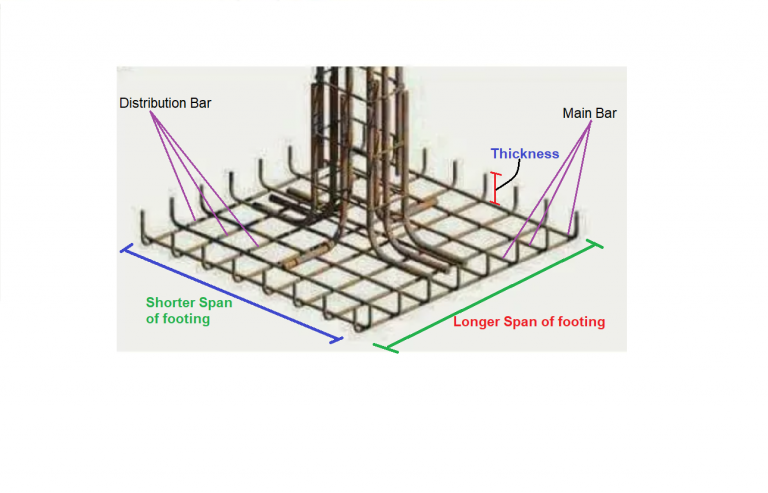Work Hours
Monday to Friday: 7AM - 7PM
Weekend: 10AM - 5PM

# Steel Weight Calculator For Footing

## Calculations for Steel Calculator for Column Footing

Let’s understand with the help of an example, how to use the above Steel Calculator for Column FootingAssumptions For Example

• The calculation procedure is for a single reinforcing net.
• There are 2 types of bars in a footing: Main Bar & Distribution Bar.
• Main Bar Diameter = 12 mm
• Spacing Between Main Bars (C/C distance) = 100 mm
• Distribution Bar Diameter = 12 mm
• Spacing Between Distribution Bars (C/C distance) = 100 mm
• Length of Footing (Longer Span) = 2 m
• Width of Footing (Shorter Span) = 2 m
• Thickness of Footing = 0.25 m
• Clear Cover from tops & sides = 50 mm
• Clear Cover from bottom= 75 mm
• We are using Steel grade of fe500.

Calculation Procedure

• Step 1: Calculation of Number of main & distribution Bar
No’s of Main bar = [(Total distance – clear cover) / Spacing] + 1
=[(2 – 0.05) / 0.1 ] + 1
= 21
No’s of Distribution bar = [(Total distance – clear cover) / Spacing] + 1
=[(2 – 0.05) / 0.1 ] + 1
= 21

• Step 2: Calculation of cutting length of main & distribution bar
Cutting Length of main bar = [Total length – 2(Half diameter of the bar + Clear cover) + 2(Thickness of the slab –Bottom and Top clear cover – Half diameter of the bar)]
=[2 – 2(0.006 + 0.05) +2(0.25 – 0.075 – 0.05 – 0.006)]
=2.12 m
Cutting Length of Distribution bar = [Total length – 2(Half diameter of the bar + Clear cover) + 2(Thickness of the slab –Bottom and Top clear cover – Diameter of the bar – Half diameter of the bar)]
=[2 – 2(0.006 + 0.05) +2(0.25 – 0.075 – 0.05 – 0.012 – 0.06)]
=2.10 m

• Step 3: Total Length Calculation
Total Length of Main Bar = 21 x 2.12
= 44.64 m
Total Length of Distribution Bar = 21 x 2.10
= 44.14 m

• Step 4: Calculation of Steel Weight
Weight of the bar as per their length and diameter by following the Formula: –
Unit weight formula for kg per metre =(D2/163) × L kg/m
Unit weight for feet per metre = (D2/ 533) × L kg/ft
L is the required length of Steel bar of Diameter D
Weight of Main Bar = (122/163) x 44.65
= 39.45 Kg
Weight of Distribution Bar = (122/163) x 44.15
= 39 Kg

Final Result

Total Steel Weight =  39.45 + 39  =78.45 Kg

Try Onsite, #1 Tool For Site Tracking

/** zohoform script */